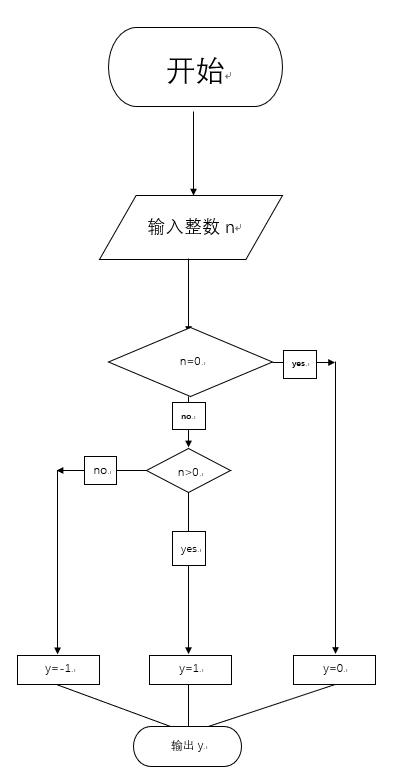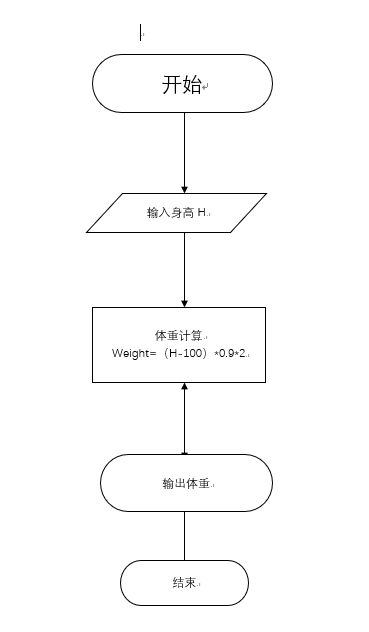输入格式:

10

sign(10) = 1

0

sign(0) = 0

-98

sign(-98) = -1
#include<stdio.h>
int main()
{
int n,y;
scanf("%d",&n);
if(n!=0){
if(n>0){
y = 1;
}
else{
y = -1;
}
}
else{
y = 0;
}
printf("sign(%d) = %d",n,y);
return 0;
}过程中没有遇到问题
输入格式：

169

124.2

#include<stdio.h>
int main()
{
int H;
float weight;
scanf("%d",&H);
weight = (H-100)*0.9*2;
printf("%.1f",weight);
return 0;

}